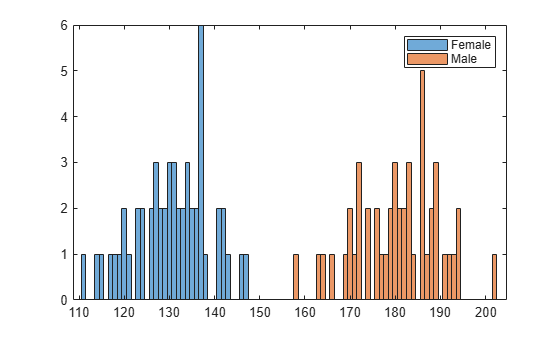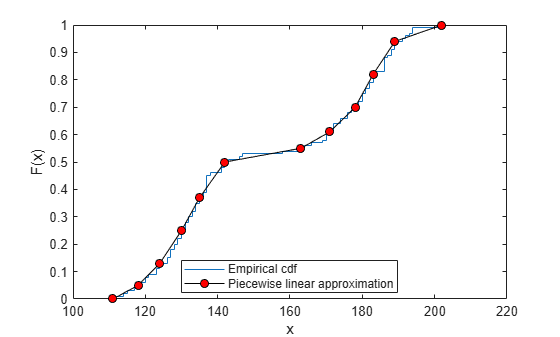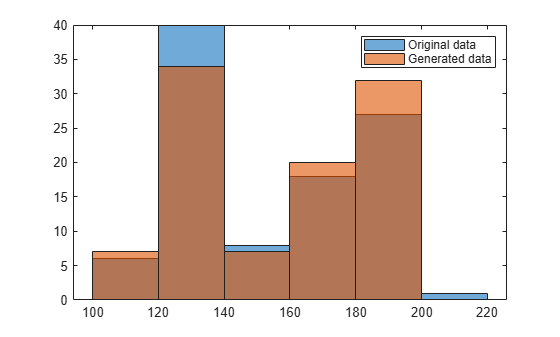# PiecewiseLinearDistribution

Piecewise linear probability distribution object

## Description

A `PiecewiseLinearDistribution` object consists of a model description for a piecewise linear probability distribution.

The piecewise linear distribution is a nonparametric probability distribution created using a piecewise linear representation of the cumulative distribution function (cdf). The options specified for the piecewise linear distribution specify the form of the cdf. The probability density function (pdf) is a step function.

The piecewise linear distribution uses the following parameters.

ParameterDescription
`x`Vector of x values at which the cdf changes slope
`Fx`Vector of cdf values that correspond to each value in `x`

## Creation

Create a `PiecewiseLinearDistribution` probability distribution with specified parameter values object using `makedist`.

## Properties

expand all

### Distribution Parameters

Data values at which the cumulative distribution function (cdf) changes slope, specified as a vector of scalar values.

Data Types: `single` | `double`

cdf value at each value in `x`, specified as a vector of scalar values.

Data Types: `single` | `double`

### Distribution Characteristics

Logical flag for truncated distribution, specified as a logical value. If `IsTruncated` equals `0`, the distribution is not truncated. If `IsTruncated` equals `1`, the distribution is truncated.

Data Types: `logical`

Number of parameters for the probability distribution, specified as a positive integer value.

Data Types: `double`

Distribution parameter values, specified as a vector of scalar values.

Data Types: `single` | `double`

Truncation interval for the probability distribution, specified as a vector of scalar values containing the lower and upper truncation boundaries.

Data Types: `single` | `double`

### Other Object Properties

Probability distribution name, specified as a character vector.

Data Types: `char`

Distribution parameter descriptions, specified as a cell array of character vectors. Each cell contains a short description of one distribution parameter.

Data Types: `char`

Distribution parameter names, specified as a cell array of character vectors.

Data Types: `char`

## Object Functions

 `cdf` Cumulative distribution function `icdf` Inverse cumulative distribution function `iqr` Interquartile range of probability distribution `mean` Mean of probability distribution `median` Median of probability distribution `pdf` Probability density function `plot` Plot probability distribution object `random` Random numbers `std` Standard deviation of probability distribution `truncate` Truncate probability distribution object `var` Variance of probability distribution

## Examples

collapse all

Create a piecewise linear distribution object using the default parameter values.

`pd = makedist('PiecewiseLinear')`
```pd = PiecewiseLinearDistribution F(0) = 0 F(1) = 1 ```

Compute the empirical cumulative distribution function (cdf) for data, and create a piecewise linear distribution object using an approximation to the empirical cdf.

Load the sample data. Visualize the patient weight data using a histogram.

```load patients histogram(Weight(strcmp(Gender,'Female'))) hold on histogram(Weight(strcmp(Gender,'Male'))) legend('Female','Male')```The histogram shows that the data has two modes, one for female patients and one for male patients.

Compute the empirical cdf for the data.

`[f,x] = ecdf(Weight);`

Construct a piecewise linear approximation to the empirical cdf by taking a value every five points.

```f = f(1:5:end); x = x(1:5:end);```

Plot the empirical cdf and the approximation.

```figure ecdf(Weight) hold on plot(x,f,'ko-','MarkerFace','r') legend('Empirical cdf','Piecewise linear approximation', ... 'Location','best')```Create a piecewise linear probability distribution object using the piecewise approximation of the empirical cdf.

`pd = makedist('PiecewiseLinear','x',x,'Fx',f)`
```pd = PiecewiseLinearDistribution F(111) = 0 F(118) = 0.05 F(124) = 0.13 F(130) = 0.25 F(135) = 0.37 F(142) = 0.5 F(163) = 0.55 F(171) = 0.61 F(178) = 0.7 F(183) = 0.82 F(189) = 0.94 F(202) = 1 ```

Generate 100 random numbers from the distribution.

```rng('default') % For reproducibility rw = random(pd,[100,1]);```

Plot the random numbers to visually compare their distribution to the original data.

```figure histogram(Weight) hold on histogram(rw) legend('Original data','Generated data')```The random numbers generated from the piecewise linear distribution have the same bimodal distribution as the original data.

## Version History

Introduced in R2013a